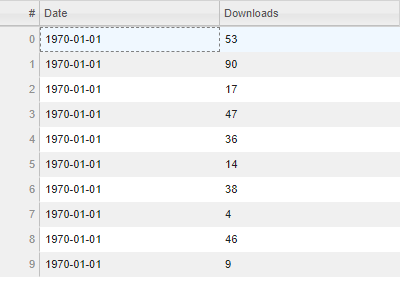# Examples for DataTable onclick event

Hi - Is there any sample code on how to create on-click event for DataTable. For example, for the below data table, when I click on any row, I would like to read that row and create another graph, etc. Appreciate any suggestions.

``````from datetime import date
from random import randint

from bokeh.io import show
from bokeh.models import ColumnDataSource, DataTable, DateFormatter, TableColumn

data = dict(
dates=[date(2014, 3, i+1) for i in range(10)],
)
source = ColumnDataSource(data)

columns = [
TableColumn(field="dates", title="Date", formatter=DateFormatter()),
]
data_table = DataTable(source=source, columns=columns, width=400, height=280)

show(data_table)
``````Hi @Chetan_Ambi ,

When you click on a row in a DataTable, you are actually changing the `selected` attribute in the ColumnDataSource behind it. The trick is to link a callback to `source.selected.js_on_change`, so that when you click on a row in the DataTable something happens. (If you’re using Bokeh server and python callbacks, then this is just `on_change`.)

Here is a small, hastily written example of using `selected.js_on_change` to show a selected row’s values in another section in the layout. You can expand upon this idea to use those values for something else, like to create a new plot, as you mention.

``````from bokeh.models import DataTable, TableColumn, ColumnDataSource, Div, CustomJS
from bokeh.layouts import row
from bokeh.plotting import show

x = [2, 4, 8, 5, 9, 3]
y = [4, 8, 2, 7, 4, 6]

cds = ColumnDataSource(data={'x': x, 'y': y})

columns = [
TableColumn(field='x', title='X'),
TableColumn(field='y', title='Y'),
]
data_table = DataTable(source=cds, columns=columns, width=300, height=400)

output_div = Div()

row = row(data_table, output_div)

callback = CustomJS(args=dict(row=row, x=x, y=y, output_div=output_div), code="""
// "index" here is the number of the row you clicked in the table.
var index = cb_obj.indices
console.log(index)
output_div.text = "(" + x[index] + "," + y[index] + ")"

// reconstruct and reassign the row's children
var children = [...row.children]
children = output_div
row.children = children
""")

cds.selected.js_on_change('indices', callback)

show(row)
``````